Notebook

# Pairs Trading with Machine LearningÂ¶

Jonathan Larkin

August, 2017

In developing a Pairs Trading strategy, finding valid, eligible pairs which exhibit unconditional mean-reverting behavior is of critical importance. This notebook walks through an example implementation of finding eligible pairs. We show how popular algorithms from Machine Learning can help us navigate a very high-dimensional seach space to find tradeable pairs.

In :
import matplotlib.pyplot as plt
import matplotlib.cm as cm

import numpy as np
import pandas as pd

from sklearn.cluster import KMeans, DBSCAN
from sklearn.decomposition import PCA
from sklearn.manifold import TSNE
from sklearn import preprocessing

from statsmodels.tsa.stattools import coint

from scipy import stats

from quantopian.pipeline.data import morningstar
from quantopian.pipeline.filters.morningstar import Q500US, Q1500US, Q3000US
from quantopian.pipeline import Pipeline
from quantopian.research import run_pipeline

In :
print "Numpy: %s " % np.__version__
print "Pandas: %s " % pd.__version__

Numpy: 1.11.1
Pandas: 0.18.1

In :
study_date = "2016-12-31"


## Define UniverseÂ¶

We start by specifying that we will constrain our search for pairs to the a large and liquid single stock universe.

In :
universe = Q1500US()


## Choose DataÂ¶

In addition to pricing, let's use some fundamental and industry classification data. When we look for pairs (or model anything in quantitative finance), it is generally good to have an "economic prior", as this helps mitigate overfitting. I often see Quantopian users create strategies with a fixed set of pairs that they have likely chosen by some fundamental rationale ("KO and PEP should be related becuase..."). A purely fundamental approach is a fine way to search for pairs, however breadth will likely be low. As discussed in The Foundation of Algo Success, you can maximize Sharpe by having high breadth (high number of bets). With N stocks in the universe, there are N*(N-1)/2 pair-wise relationships. However, if we do a brute-force search over these, we will likely end up with many spurious results. As such, let's narrow down the search space in a reasonable way. In this study, I start with the following priors:

• Stocks that share loadings to common factors (defined below) in the past should be related in the future.
• Stocks of similar market caps should be related in the future.
• We should exclude stocks in the industry group "Conglomerates" (industry code 31055). Morningstar analysts classify stocks into industry groups primarily based on similarity in revenue lines. "Conglomerates" is a catch-all industry. As described in the Morningstar Global Equity Classification Structure manual: "If the company has more than three sources of revenue and income and there is no clear dominant revenue and income stream, the company is assigned to the Conglomerates industry." We should not expect these stocks to be good members of any pairs in the future. This turns out to have zero impact on the Q500 and removes only 1 stock from the Q1500, but I left this idea in for didactic purposes.
• Creditworthiness in an important feature in future company performance. It's difficult to find credit spread data and map the reference entity to the appropriate equity security. There is a model, colloquially called the Merton Model, however, which takes a contingent claims approach to modeling the capital structure of the firm. The output is an implied probability of default. Morningstar analysts calculate this for us and the field is called financial_health_grade. A full description of this field is in the help docs.
In :
pipe = Pipeline(
columns= {
'Market Cap': morningstar.valuation.market_cap.latest.quantiles(5),
'Industry': morningstar.asset_classification.morningstar_industry_group_code.latest,
},
screen=universe
)

In :
res = run_pipeline(pipe, study_date, study_date)
res.index = res.index.droplevel(0)  # drop the single date from the multi-index

In :
print res.shape

(1500, 3)

Out:
Financial Health Industry Market Cap
Equity(2 [ARNC]) C 10106 4
Equity(24 [AAPL]) A 31167 4
Equity(52 [ABM]) B 31054 3
Equity(53 [ABMD]) A 20639 3
Equity(62 [ABT]) B 20639 4
In :
# remove stocks in Industry "Conglomerates"
res = res[res['Industry']!=31055]
print res.shape

(1499, 3)

In :
# remove stocks without a Financial Health grade
res = res[res['Financial Health']!= None]
print res.shape

(1499, 3)

In :
# replace the categorical data with numerical scores per the docs
res['Financial Health'] = res['Financial Health'].astype('object')
health_dict = {u'A': 0.1,
u'B': 0.3,
u'C': 0.7,
u'D': 0.9,
u'F': 1.0}
res = res.replace({'Financial Health': health_dict})

In :
res.describe()

Out:
Financial Health Industry Market Cap
count 1499.000000 1499.000000 1499.000000
mean 0.447432 20262.618412 3.377585
std 0.266508 9205.899868 0.654800
min 0.100000 10101.000000 2.000000
25% 0.300000 10320.000000 3.000000
50% 0.300000 20635.000000 3.000000
75% 0.700000 31054.000000 4.000000
max 1.000000 31169.000000 4.000000

## Define HorizonÂ¶

We are going to work with a daily return horizon in this strategy.

In :
pricing = get_pricing(
symbols=res.index,
fields='close_price',
start_date=pd.Timestamp(study_date) - pd.DateOffset(months=24),
end_date=pd.Timestamp(study_date)
)

In :
pricing.shape

Out:
(505, 1499)
In :
returns = pricing.pct_change()

In :
returns[symbols(['AAPL'])].plot();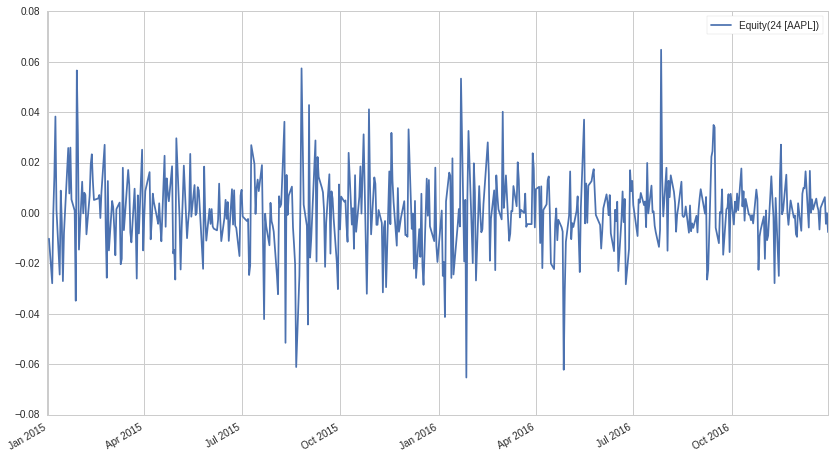In :
# we can only work with stocks that have the full return series
returns = returns.iloc[1:,:].dropna(axis=1)

In :
print returns.shape

(504, 1429)


## Find Candidate PairsÂ¶

Given the pricing data and the fundamental and industry/sector data, we will first classify stocks into clusters and then, within clusters, looks for strong mean-reverting pair relationships.

The first hypothesis above is that "Stocks that share loadings to common factors in the past should be related in the future". Common factors are things like sector/industry membership and widely known ranking schemes like momentum and value. We could specify the common factors a priori to well known factors, or alternatively, we could let the data speak for itself. In this post we take the latter approach. We use PCA to reduce the dimensionality of the returns data and extract the historical latent common factor loadings for each stock. For a nice visual introduction to what PCA is doing, take a look here (thanks to Gus Gordon for pointing out this site).

We will take these features, add in the fundamental features, and then use the DBSCAN unsupervised clustering algorithm which is available in scikit-learn. Thanks to Thomas Wiecki for pointing me to this specific clustering technique and helping with implementation. Initially I looked at using KMeans but DBSCAN has advantages in this use case, specifically

• DBSCAN does not cluster all stocks; it leaves out stocks which do not neatly fit into a cluster;
• relatedly, you do not need to specify the number of clusters.

The clustering algorithm will give us sensible candidate pairs. We will need to do some validation in the next step.

### PCA Decomposition and DBSCAN ClusteringÂ¶

In :
N_PRIN_COMPONENTS = 50
pca = PCA(n_components=N_PRIN_COMPONENTS)
pca.fit(returns)

Out:
PCA(copy=True, n_components=50, whiten=False)
In :
pca.components_.T.shape

Out:
(1429, 50)

We have reduced data now with the first N_PRIN_COMPONENTS principal component loadings. Let's add some fundamental values as well to make the model more robust.

In :
X = np.hstack(
(pca.components_.T,
res['Market Cap'][returns.columns].values[:, np.newaxis],
res['Financial Health'][returns.columns].values[:, np.newaxis])
)

print X.shape

(1429, 52)

In :
X = preprocessing.StandardScaler().fit_transform(X)
print X.shape

(1429, 52)

In :
clf = DBSCAN(eps=1.9, min_samples=3)
print clf

clf.fit(X)
labels = clf.labels_
n_clusters_ = len(set(labels)) - (1 if -1 in labels else 0)
print "\nClusters discovered: %d" % n_clusters_

clustered = clf.labels_

DBSCAN(algorithm='auto', eps=1.9, leaf_size=30, metric='euclidean',
min_samples=3, p=None, random_state=None)

Clusters discovered: 11

In :
# the initial dimensionality of the search was
ticker_count = len(returns.columns)
print "Total pairs possible in universe: %d " % (ticker_count*(ticker_count-1)/2)

Total pairs possible in universe: 1020306

In :
clustered_series = pd.Series(index=returns.columns, data=clustered.flatten())
clustered_series_all = pd.Series(index=returns.columns, data=clustered.flatten())
clustered_series = clustered_series[clustered_series != -1]

In :
CLUSTER_SIZE_LIMIT = 9999
counts = clustered_series.value_counts()
ticker_count_reduced = counts[(counts>1) & (counts<=CLUSTER_SIZE_LIMIT)]
print "Clusters formed: %d" % len(ticker_count_reduced)
print "Pairs to evaluate: %d" % (ticker_count_reduced*(ticker_count_reduced-1)).sum()

Clusters formed: 11
Pairs to evaluate: 2120


We have reduced the search space for pairs from >1mm to approximately 2,000.

### Cluster VisualizationÂ¶

We have found 11 clusters. The data are clustered in 52 dimensions. As an attempt to visualize what has happened in 2d, we can try with T-SNE. T-SNE is an algorithm for visualizing very high dimension data in 2d, created in part by Geoff Hinton. We visualize the discovered pairs to help us gain confidence that the DBSCAN output is sensible; i.e., we want to see that T-SNE and DBSCAN both find our clusters.

In :
X_tsne = TSNE(learning_rate=1000, perplexity=25, random_state=1337).fit_transform(X)

In :
plt.figure(1, facecolor='white')
plt.clf()
plt.axis('off')

plt.scatter(
X_tsne[(labels!=-1), 0],
X_tsne[(labels!=-1), 1],
s=100,
alpha=0.85,
c=labels[labels!=-1],
cmap=cm.Paired
)

plt.scatter(
X_tsne[(clustered_series_all==-1).values, 0],
X_tsne[(clustered_series_all==-1).values, 1],
s=100,
alpha=0.05
)

plt.title('T-SNE of all Stocks with DBSCAN Clusters Noted');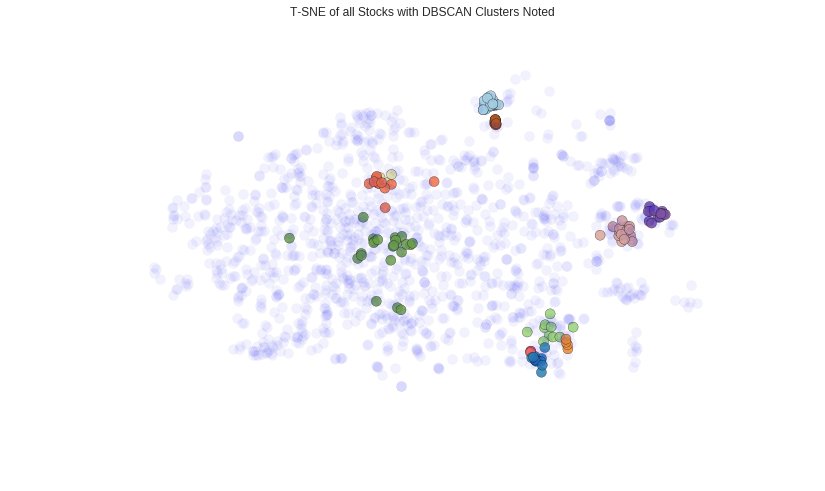We can also see how many stocks we found in each cluster and then visualize the normalized time series of the members of a handful of the smaller clusters.

In :
plt.barh(
xrange(len(clustered_series.value_counts())),
clustered_series.value_counts()
)
plt.title('Cluster Member Counts')
plt.xlabel('Stocks in Cluster')
plt.ylabel('Cluster Number');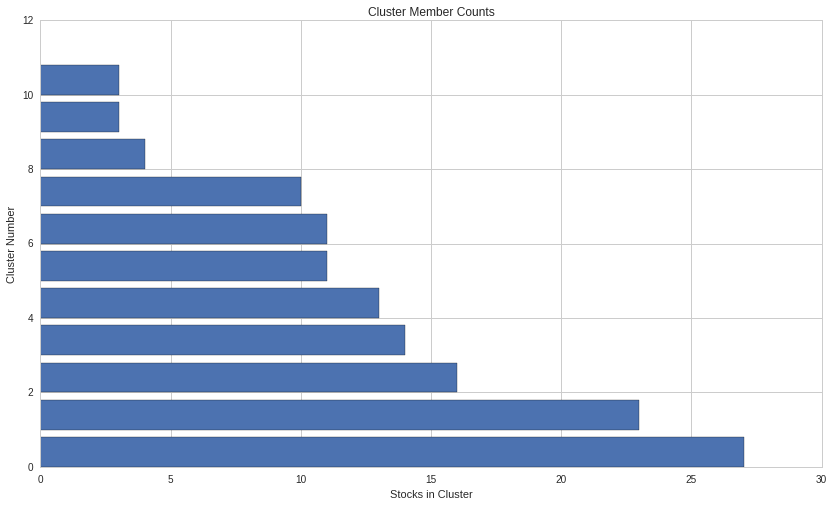To again visualize if our clustering is doing anything sensible, let's look at a few clusters (for reproducibility, keep all random state and dates the same in this notebook).

In :
# get the number of stocks in each cluster
counts = clustered_series.value_counts()

# let's visualize some clusters
cluster_vis_list = list(counts[(counts<20) & (counts>1)].index)[::-1]

# plot a handful of the smallest clusters
for clust in cluster_vis_list[0:min(len(cluster_vis_list), 3)]:
tickers = list(clustered_series[clustered_series==clust].index)
means = np.log(pricing[tickers].mean())
data = np.log(pricing[tickers]).sub(means)
data.plot(title='Stock Time Series for Cluster %d' % clust)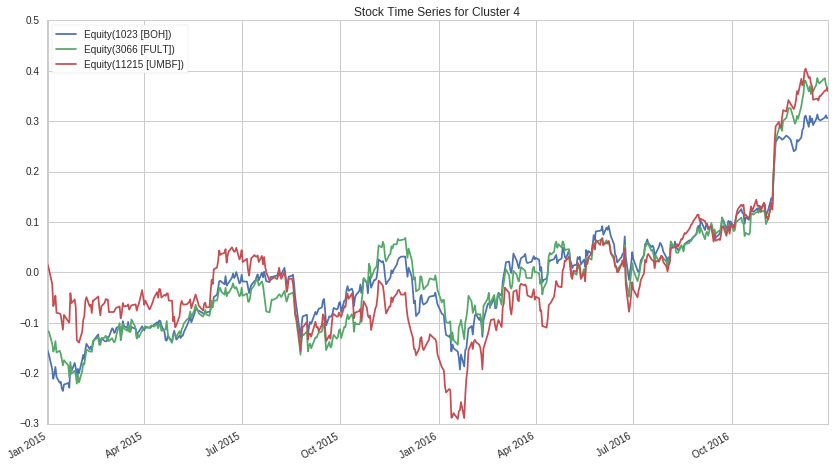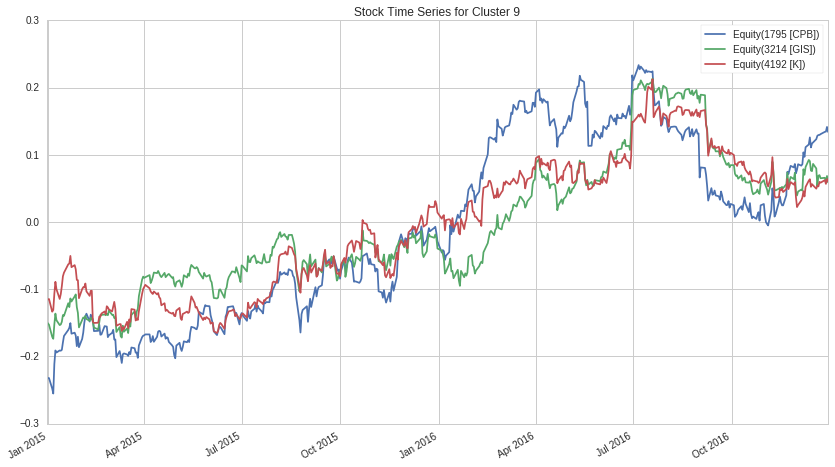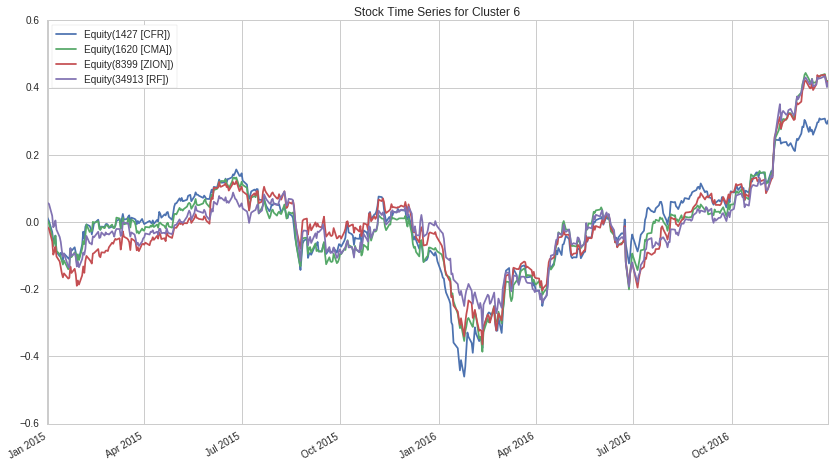We might be interested to see how a cluster looks for a particular stock. Large bank stocks share similar strict regulatory oversight and are similarly economic and interest rate sensitive. We indeed see that our clustering has found a bank stock cluster.

In :
which_cluster = clustered_series.loc[symbols('JPM')]
clustered_series[clustered_series == which_cluster]

Out:
Equity(903 [BK])       2
Equity(5117 [MTB])     2
Equity(5479 [NTRS])    2
Equity(5769 [PBCT])    2
Equity(7152 [STI])     2
Equity(8151 [WFC])     2
Equity(16850 [BBT])    2
Equity(20088 [GS])     2
Equity(25006 [JPM])    2
Equity(25010 [USB])    2
dtype: int64
In :
tickers = list(clustered_series[clustered_series==which_cluster].index)
means = np.log(pricing[tickers].mean())
data = np.log(pricing[tickers]).sub(means)
data.plot(legend=False, title="Stock Time Series for Cluster %d" % which_cluster);